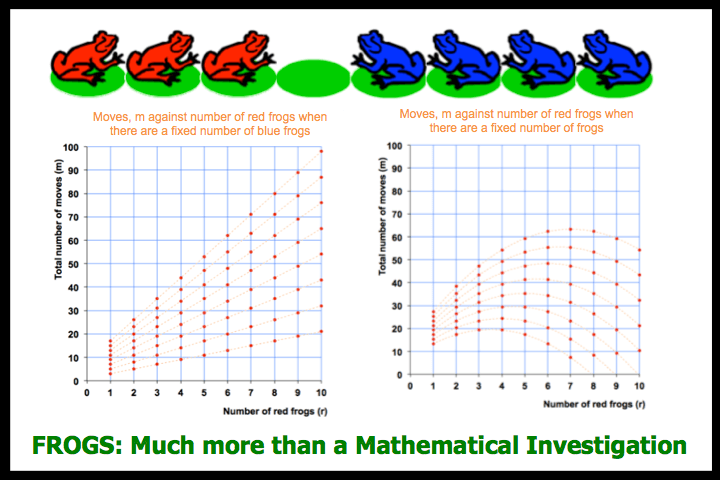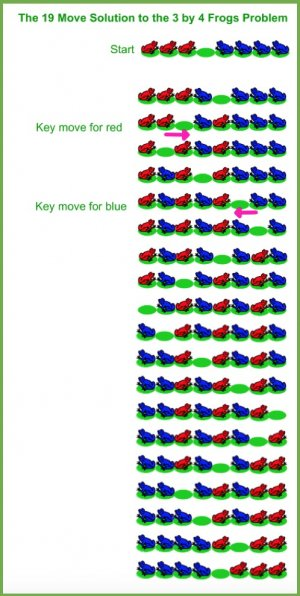Frogs – a pdf file that gives background, how to solve the frogs problem, how to link it to solving quadratics, graph and formula work, where to find a proof as well as hints to the proof.

Frogs – a spreadsheet that can be used interactively to demonstrate the problem and complete activities noted in pdf file above.

Frogs – ActivInspire IWB file for pdf and spreadsheet files above.

Frogs – Original Sources for the problem

### Virtual Manipulatives

Two Frogs Virtual Manipulatives are given below (a main lesson and plenary following from it): they will not work on iPhones or iPads since they are flash files. They have some links to the resources above.Frogs: main lesson flash file

Lesson Notes for aboveFrogs: lesson plenary flash file

Lesson Notes for above

The book below is one of the places where this activity can be found.Mathematical Recreations and Essays (Dover Recreational Math)Solution to the Above Problem